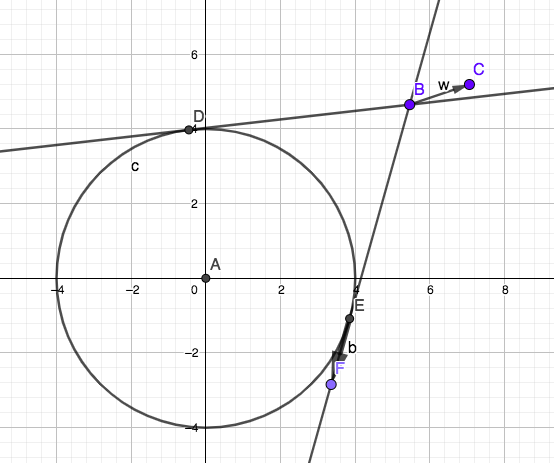# Finding a tangent vector from a given point and circle

Hi all

Im working on some AI navigation, and I would like the vehicle to aim up for the tangent b (reference picture at the bottom). I got most of the stuff figured out at the moment (thanks to this great answer), but I’m still struggling with the maths in C#.

I have the point B, the direction of vector w, the length shouldn’t matter.
I also have point A, and the radius of the circle. What I’m missing is the point E, which is the intersecting point between the tangent of the circle, and the circle itself, and the direction of vector b.

Approximations are also alright, if they grant a performance boost - I’m not looking for accuracy, just a decent behaving aiAll help is appreciated!oh hey, i know this.

core routine:

``````  // this approach is more geometrical and less algebraic than approach 1,
// and far more stable. thanks to Mike Plotz for suggesting this direction.
bool CircleTangents_2(Vector2 center, float r, Vector2 p, ref Vector2 tanPosA, ref Vector2 tanPosB) {
p -= center;

float P = p.magnitude;

// if p is inside the circle, there ain't no tangents.
if (P <= r) {
return false;
}

float a = r * r                                          / P;
float q = r * (float)System.Math.Sqrt((P * P) - (r * r)) / P;

Vector2 pN  = p / P;
Vector2 pNP = new Vector2(-pN.y, pN.x);
Vector2 va  = pN * a;

tanPosA = va + pNP * q;
tanPosB = va - pNP * q;

tanPosA += center;
tanPosB += center;

return true;
}
``````

and finally a picture: